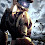Programming Geek
Rated 4.7/5 based on 1446 reviews### Kosaraju's Algorithm to Find Strongly Connected Components

Have you ever thought how Facebook suggests you groups to join or pages to like or even  "people you may know" . If not then have a look here. In fact Facebook uses graph to represent people. You can visit  http://graph.facebook.com/vikashvverma to have a look how each individual is represented on Facebook. This is the beautiful application of Graph on social networking sites. Let us hve a look on the same.

A graph is said to be strongly connected if there is a path from each vertex to every other vertex i.e if u and v are two vertices then there is a path from u to v and also from v to u.  One of the algorithm to compute the strongly connected components of a graph is due to Kosaraju ; a bachelor of engineering from  Andhra University, masters from IIT Kharagpur and PhD from  University of Pennsylvania. He used Depth First Search of Graph to find strongly connected components of the graph in O(m+n) time.The strongly connected component of a graph is shown below (The vertices inside the colored part shows strongly connected components )  :

Algorithm :

1) G is a directed graph and S is a stack.

2) While S does not contain all vertices perform step 3.

3)choose a random vertex v and perform depth first search on it. Each time DFS finishes expanding vertex v, push v on to the stack S. (This guarantees that the vertex with maximum finish time will more closer to the top of the stack).

4) Obtain a transpose of the G by reversing the direction of the edge.

5)While S is not empty perform step 6.

6) Remove v=top of S and again perform DFS on it The set of all visited vertices will give the strongly connected components containing v. Remove all visited vertices from stack.

Here goes my JAVA implementation of Kosaraju's Algorithm to find strongly connected components . The program reads data from in.txt file having first column as tail and second column as head. For more verices node value should be changed.

``````import java.io.File;
import java.util.ArrayList;
import java.util.Arrays;
import java.util.Collections;
import java.util.Scanner;
import java.util.Stack;

/**
*
*@author Vikash VIK
*/
public class SCC {
static Stack<Integer> st = new Stack<Integer>();
final static int node=5;//for more vertices reset this value
static boolean visited[] = new boolean[node];
public static void main(String[] args)throws Exception {

process();
}
public static void process()throws Exception  {

for (int i = 0; i < visited.length; i++) {
}
Scanner sc = new Scanner(new File("./in.txt"));
while (sc.hasNextLine()) {
String[] s = sc.nextLine().trim().split(" ");
LinkedList<Integer> list = ls.get(Integer.parseInt(s.trim()) - 1);
}
for (int i = 0; i < visited.length; i++) {
if (visited[i] == false) {
DFS(i + 1);
}
}
for (int i = 0; i < visited.length; i++) {
visited[i] = false;
}
for (int i = 0; i < visited.length; i++) {
}
sc = new Scanner(new File("./in.txt"));
while (sc.hasNextLine()) {
String[] s = sc.nextLine().trim().split(" ");
LinkedList<Integer> list = ls.get(Integer.parseInt(s.trim()) - 1);

}
System.out.println("Strongly connected components are : ");
while (st.empty() == false) {
System.out.println();
DFS1(st.pop());
}

}
public static void DFS(int v)  {
visited[v - 1] = true;
LinkedList<Integer> list = ls.get(v - 1);
while (!(list.isEmpty())) {
int temp = list.removeFirst();

if (visited[temp - 1] == false) {
DFS(temp);
}

}
st.push(v);
}

public static void DFS1(int v)  {
visited[v - 1] = true;
System.out.print(v + "\t");
st.remove(new Integer(v));
LinkedList<Integer> list = ls.get(v - 1);
while (!list.isEmpty()) {
int temp = list.removeFirst();
if (visited[temp - 1] == false) {
DFS1(temp);
}
}
}

}```
```

Hence stronly connected components find common interests of a group of people and suggests them group or pages or people. Isn't it a great apllication of graph in social networking sites.

1.1.